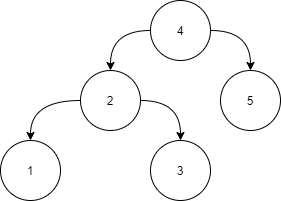Validate BST

Posted: 20 Oct, 2020
Difficulty: Moderate

PROBLEM STATEMENT

A binary search tree (BST) is a binary tree data structure which has the following properties.

• The left subtree of a node contains only nodes with data less than the node’s data.
• The right subtree of a node contains only nodes with data greater than the node’s data.
• Both the left and right subtrees must also be binary search trees.

Input:Level 1:

All the nodes in the left subtree of 4 (2, 1, 3) are smaller
than 4, all the nodes in the right subtree of the 4 (5) are
larger than 4.

Level 2 :

For node 2:
All the nodes in the left subtree of 2 (1) are smaller than
2, all the nodes in the right subtree of the 2 (3) are larger than 2.
For node 5:
The left and right subtree for node 5 is empty.

Level 3:

For node 1:
The left and right subtree for node 1 are empty.
For node 3:
The left and right subtree for node 3 are empty.
Because all the nodes follow the property of a binary
search tree, the above tree is a binary search tree.
Input Format:
The first line contains an Integer 't', which denotes the number of test cases or queries to be run. Then the test cases follow.

The first line of input contains the elements of the tree in the level order form separated by a single space.
If any node does not have a left or right child, take -1 in its place. Refer to the example below.

Example:

Elements are in the level order form. The input consists of values of nodes separated by a single space in a single line. In case a node is null, we take -1 in its place.

For example, the input for the tree depicted in the below image would be :1
2 3
4 -1 5 6
-1 7 -1 -1 -1 -1
-1 -1

Explanation :
Level 1 :
The root node of the tree is 1

Level 2 :
Left child of 1 = 2
Right child of 1 = 3

Level 3 :
Left child of 2 = 4
Right child of 2 = null (-1)
Left child of 3 = 5
Right child of 3 = 6

Level 4 :
Left child of 4 = null (-1)
Right child of 4 = 7
Left child of 5 = null (-1)
Right child of 5 = null (-1)
Left child of 6 = null (-1)
Right child of 6 = null (-1)

Level 5 :
Left child of 7 = null (-1)
Right child of 7 = null (-1)

The first not-null node (of the previous level) is treated as the parent of the first two nodes of the current level. The second not-null node (of the previous level) is treated as the parent node for the next two nodes of the current level and so on.

The input ends when all nodes at the last level are null (-1).
Note :
The above format was just to provide clarity on how the input is formed for a given tree.

The sequence will be put together in a single line separated by a single space. Hence, for the above-depicted tree, the input will be given as:

1 2 3 4 -1 5 6 -1 7 -1 -1 -1 -1 -1 -1
Output Format :
For each test case, return true if the binary tree is a BST, else return false.

Output for every test case will be denoted in a separate line.
Note:
You are not required to print anything explicitly. It has already been taken care of. Just implement the function.
Constraint :
1 <= T <= 100
1 <= N <= 1000
-10^6 <= data <= 10^6
where N is the number of nodes in the tree, T represents the number of test cases, and data denotes data contained in the node of the binary tree.

Duplicate elements can be in the right subtree or left subtree.

Time Limit: 1 secApproach 1
• For each node, store the minimum and maximum value allowed for that node.
• Initially, for the root node as all the integer values are allowed, the minimum value would be -10^9 and the maximum value should be 10^9. Here we can also use built-in INT_MIN and INT_MAX constants.
• If the value of that node is not in the bounded range of minimum and maximum value, then return false.
• For the left subtree of a node with data ‘x’, update the maximum value to ‘x’.
• For the right subtree of a node with data ‘x’, update the minimum value to ‘x’.
• This will make sure every node in the left subtree will be less than or equal to x and every node in the right subtree will be greater than or equal to x.
• Check this for each node of the tree, if every node is in the range then the tree is a binary search tree.